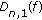# A New Estimate on the Rate of Convergence of Durrmeyer-Bézier Operators

## Abstract

We obtain an estimate on the rate of convergence of Durrmeyer-Bézier operaters for functions of bounded variation by means of some probabilistic methods and inequality techniques. Our estimate improves the result of Zeng and Chen (2000).

## 1. Introdution

In 2000, Zeng and Chen  introduced the Durrmeyer-Bézier operatorswhich are defined as follows:(1.1)

whereis defined on,,,,are Bézier basis functions, and,are Bernstein basis functions.

When,is just the well-known Durrmeyer operator(1.2)

Concerning the approximation properties of operatorsand some results on approximation of functions of bounded variation by positive linear operators, one can refer to . Authors of  studied the rate of convergence of the operatorsfor functions of bounded variation and presented the following important result.

Theorem A

Letbe a function of bounded variation on, (),then for everyandone has(1.3)

whereis the total variation ofonand(1.4)

Since the Durrmeyer-Bézier operatorsare an important approximation operator of new type, the purpose of this paper is to continue studying the approximation properties of the operatorsfor functions of bounded variation, and give a better estimate than that of Theorem A by means of some probabilistic methods and inequality techniques. The result of this paper is as follows.

Theorem 1.1.

Letbe a function of bounded variation on, (),then for everyandone has(1.5)

whereis defined in (1.4).

It is obvious that the estimate (1.5) is better than the estimate (1.3). More important, the estimate (1.5) is true for all. This is an important improvement comparing with the fact that estimate (1.3) holds only for.

## 2. Some Lemmas

In order to prove Theorem 1.1, we need the following preliminary results.

Lemma 2.1.

Letbe a sequence of independent and identically distributed random variables,is a random variable with two-point distribution(andis a parameter). Setwith the mathematical expectationand with the varianceThen forone has(2.1)(2.2)

Proof.

Since, from the distribution series of, by convolution computation we get(2.3)

Furthermore by direct computations we have(2.4)

Thus we deduce that(2.5)

By Schwarz's inequality, it follows that(2.6)

The inequality (2.1) is proved.

Similarly, by using the identities(2.7)

we get the inequality (2.2). Lemma 2.1 is proved.

Lemma 2.2.

Let,be Bernstein basis functions, and letbe Bézier basis functions, then one has(2.8)

Proof.

Note that, andThus(2.9)

Now by inequality (2.1) of Lemma 2.1 we obtain(2.10)

Similarly, by using inequality (2.2), we obtain(2.11)

Thus Lemma 2.2 is proved.

## 3. Proof of Theorem 1.1

Letsatisfy the conditions of Theorem 1.1, thencan be decomposed as(3.1)

where(3.2)

Obviouslythus from (3.1) we get(3.3)

We first estimate, from [1, page 11] we have the following equation:(3.4)

where.

Thus by Lemma 2.2, we get. Note that, we have(3.5)

Next we estimate. From (15) of , it follows the inequality(3.6)

That is,(3.7)

On the other hand, note that, we have(3.8)

From (3.7) and (3.8) we obtain(3.9)

Using inequality(3.10)

we get(3.11)

Thus from (3.9) we obtain(3.12)

Theorem 1.1 now follows by collecting the estimations (3.3), (3.5), and (3.12).

## References

1. Zeng X-M, Chen W: On the rate of convergence of the generalized Durrmeyer type operators for functions of bounded variation. Journal of Approximation Theory 2000,102(1):1–12. 10.1006/jath.1999.3367

2. Bojanić R, Chêng FH: Rate of convergence of Bernstein polynomials for functions with derivatives of bounded variation. Journal of Mathematical Analysis and Applications 1989,141(1):136–151. 10.1016/0022-247X(89)90211-4

3. Derriennic MM: Sur l'approximation de fonctions intégrables sur [0, 1] par des polynômes de Bernstein modifies. Journal of Approximation Theory 1981,31(4):325–343. 10.1016/0021-9045(81)90101-5

4. Guo SS: On the rate of convergence of the Durrmeyer operator for functions of bounded variation. Journal of Approximation Theory 1987,51(2):183–192. 10.1016/0021-9045(87)90033-5

5. Gupta V, Pant RP: Rate of convergence for the modified Szász-Mirakyan operators on functions of bounded variation. Journal of Mathematical Analysis and Applications 1999,233(2):476–483. 10.1006/jmaa.1999.6289

6. Shiryayev AN: Probability, Graduate Texts in Mathematics. Volume 95. Springer, New York, NY, USA; 1984:xi+577.

7. Zeng X-M, Gupta V: Rate of convergence of Baskakov-Bézier type operators for locally bounded functions. Computers & Mathematics with Applications 2002,44(10–11):1445–1453. 10.1016/S0898-1221(02)00269-9

## Acknowledgment

The present work is supported by Project 2007J0188 of Fujian Provincial Science Foundation of China.

## Author information

Authors

### Corresponding author

Correspondence to Pinghua Wang.

## Rights and permissions

Reprints and Permissions

Wang, P., Zhou, Y. A New Estimate on the Rate of Convergence of Durrmeyer-Bézier Operators. J Inequal Appl 2009, 702680 (2009). https://doi.org/10.1155/2009/702680

• Accepted:

• Published:

• DOI: https://doi.org/10.1155/2009/702680

### Keywords

• Basis Function
• Total Variation
• Direct Computation
• Probabilistic Method
• Mathematical Expectation